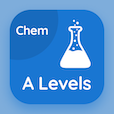Engineering Online Courses

Engineering Physics MCQs

Engineering Physics MCQ PDF - Topics

# First Law of Thermodynamics MCQ Quiz Online

Learn First Law of Thermodynamics Multiple Choice Questions (MCQ), First Law of Thermodynamics quiz answers PDF to study engineering physics online course for engineering physics classes. First Law of Thermodynamics Multiple Choice Questions and Answers (MCQs), First Law of Thermodynamics quiz questions for online colleges enrolling. "First Law of Thermodynamics MCQ" PDF Book: thermal expansion, absorption of heat by solids and liquids, heat of transformation, zeroth law of thermodynamics test prep for grad school interview questions.

"1st law of thermodynamic states that internal energy of system tends to increase if energy is" MCQ PDF: first law of thermodynamics with choices subtracted as heat, added as heat, added as electrons, and constant for online colleges enrolling. Study first law of thermodynamics quiz questions for merit scholarship test and certificate programs for graduate school interview questions.

## MCQs on First Law of Thermodynamics Quiz

MCQ: 1st law of thermodynamic states that internal energy of system tends to increase if energy is

subtracted as heat
constant

MCQ: Constant volume process is also called

isochoric process
skew rate process
free expansion process

MCQ: A process that occurs so rapid in a system that no transfer of energy as heat occurs between the system and its environment is called

constant volume
closed cycle
free expansion

MCQ: Thermodynamic process during which the volume of the closed system undergoing such a process remains constant is called

isothermic process
closed cycle process
free expansion process

MCQ: Isothermic process is also known as

isovolumetric process
closed cycle
free expansion

### More Topics from Engineering Physics Course